Courses

# Test: Electromagnetic Induction (Competition Level 2)

## 25 Questions MCQ Test Physics Class 12 | Test: Electromagnetic Induction (Competition Level 2)

Description
This mock test of Test: Electromagnetic Induction (Competition Level 2) for Class 12 helps you for every Class 12 entrance exam. This contains 25 Multiple Choice Questions for Class 12 Test: Electromagnetic Induction (Competition Level 2) (mcq) to study with solutions a complete question bank. The solved questions answers in this Test: Electromagnetic Induction (Competition Level 2) quiz give you a good mix of easy questions and tough questions. Class 12 students definitely take this Test: Electromagnetic Induction (Competition Level 2) exercise for a better result in the exam. You can find other Test: Electromagnetic Induction (Competition Level 2) extra questions, long questions & short questions for Class 12 on EduRev as well by searching above.
QUESTION: 1

Solution:
QUESTION: 2

Solution:
QUESTION: 3

### An electric current i1 can flow either direction through loop (1) and induced current i2 in loop (2). Positive i1 is when current is from `a' to `b' in loop (1) and positive i2 is when the current is from `c' to `d' in loop (2)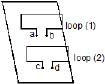In an experiment, the graph of i2 against time `t' is as shown below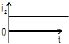Which one(s) of the following graphs could have caused i2 to behave as give above.

Solution:
QUESTION: 4

Figure shows a bar magnet and a long straight wire W, carrying current into the plane of paper. Point P is the point of intersection of axis of magnet and the line of shortest distance between magnet and the wire. If p is the midpoint of the magnet, then which of the following statements is correct ?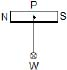Solution:
QUESTION: 5

A negative charge is given to a nonconducting loop and the loop is rotated in the plane of paper about its centre as shown in figure. The magnetic field produced by the ring affects a small magnet placed above the ring in the same plane :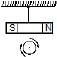Solution:
*Multiple options can be correct
QUESTION: 6

Figure shown plane figure made of a conductor located in a magnetic field along the inward normal to the plane of the figure. The magnetic field starts diminishing. Then the induced current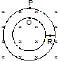Solution:
*Multiple options can be correct
QUESTION: 7

A conducting wire frame is placed in a magnetic field which is directed into the paper. The magnetic field is increasing at a constant rate. The directions of induced currents in wires AB and CD are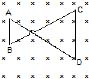Solution:
*Multiple options can be correct
QUESTION: 8

A bar magnet is moved along the axis of copper ring placed far away from the magnet. Looking from the side of the magnet, an anticlockwise current is found to be induced in the ring. Which of the following may be true?

Solution:
*Multiple options can be correct
QUESTION: 9

Two circular coils P & Q are fixed coaxially & carry currents I1 and Irespectively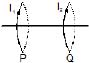Solution:
*Multiple options can be correct
QUESTION: 10

Which of the following quantities can be written in SI units in K gm2 A-2 S-3 ?

Solution:
*Multiple options can be correct
QUESTION: 11

AB and CD are smooth parallel rails, separated by a distance l, and inclined to the horizontal at an angle q. A uniform magnetic field of magnitude B, directed vertically upwards, exists in the region. EF is a conductor of mass m, carrying a current i. For EF to be in equilibrium,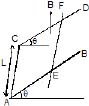Solution:
*Multiple options can be correct
QUESTION: 12

AB and CD are smooth parallel rails, separated by a distance l, and inclined to the horizontal at an angle q. A uniform magnetic field of magnitude B, directed vertically upwards, exists in the region. EF is a conductor of mass m, carrying a current i. if B is normal to the plane of the railsSolution:
*Multiple options can be correct
QUESTION: 13

A conducting rod PQ of length L = 1.0 m is moving with a uniform speed v = 20 m/s in a uniform magnetic field B = 4.0 T directed into the paper. A capacitor of capacity C = 10 mF is connected as shown in figure. Then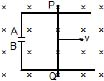Solution:
*Multiple options can be correct
QUESTION: 14

two different arrangements in which two square wire frames of same resistance are placed in a uniform constantly decreasing magnetic field B.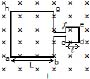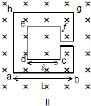The value of magnetic flux in each case is given by

Solution:
QUESTION: 15

Two different arrangements in which two square wire frames of same resistance are placed in a uniform constantly decreasing magnetic field B.The direction of induced current in the case I is

Solution:
*Multiple options can be correct
QUESTION: 16

Two different arrangements in which two square wire frames of same resistance are placed in a uniform constantly decreasing magnetic field B.The direction of induced current in the case II is

Solution:
*Multiple options can be correct
QUESTION: 17

Two different arrangements in which two square wire frames of same resistance are placed in a uniform constantly decreasing magnetic field B.Solution:
*Multiple options can be correct
QUESTION: 18

A conducting ring of radius a is rotated about a point O on its periphery as shown in the figure in a plane perpendicular to uniform magnetic field B which exists everywhere. The rotational velocity is w.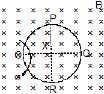Choose the correct statement(s) related to the potential of the points P, Q and R

Solution:
*Multiple options can be correct
QUESTION: 19

A conducting ring of radius a is rotated about a point O on its periphery as shown in the figure in a plane perpendicular to uniform magnetic field B which exists everywhere. The rotational velocity is w.Solution:
*Multiple options can be correct
QUESTION: 20

A conducting ring of radius a is rotated about a point O on its periphery as shown in the figure in a plane perpendicular to uniform magnetic field B which exists everywhere. The rotational velocity is w.Choose the correct statement(s) related to the induced current in ring

Solution:
*Answer can only contain numeric values
QUESTION: 21

The horizontal component of the earth's magnetic field at a place is 3 × 10-4 T and the dip is tan-1(4/3). A metal rod of length 0.25 m placed in the north-south position is moved at a constant speed of 10 cm/s towards the east. Find the e.m.f induced in the rod.

Solution:
QUESTION: 22

A wire forming one cycle of sine curve is moved in x-y plane with velocity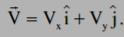. There exist a magnetic field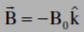. Find the motional emf develop across the ends PQ of wire.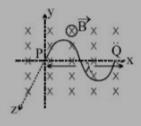Solution:
*Answer can only contain numeric values
QUESTION: 23

A conducting circular loop is placed in a uniform magnetic field of 0.02 T, with its plane perpendicular to the field. If the radius of the loop starts shrinking at a constant rate of 1.0 mm/s, then find the emf induced in the loop, at the instant when the radius is 4 cm.

Solution:
QUESTION: 24

Find the dimension of the quantity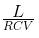, where symbols have usual meaning.

Solution:
*Answer can only contain numeric values
QUESTION: 25

A rectangular loop with a sliding connector of length l = 1.0 m is situated in a uniform magnetic field B = 2T perpendicular to the plane of loop. Resistance of connector is r = 2W. Two resistances of 6W and 3W are connected as shown in figure. Find the external force required to keep the connector moving with a constant velocity v = 2m/s.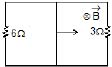Solution: